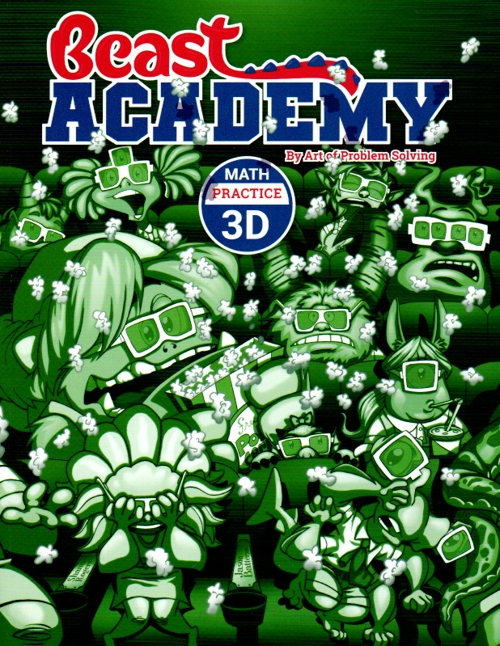Art of Problem Solving Beast Academy 3D Guide and Practice \$30 : Fractions: Unit fractions, fractions on the number line, mixed numbers, equivalent fractions, parts of a whole, comparing and ordering fractions, simplifying fractions. Estimation: Rounding, computing estimates, over and underestimating, eyeball estimates, Fermi problems. Area: Square units, adding and subtracting areas, tangrams, triangle area, unit conversions.